## The Root Test

Since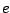and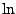are inverses, we have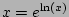. This implies the very useful fact that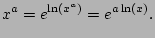As a sample application, notice that for any nonzero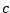,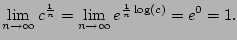Similarly,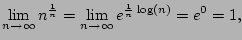where we've used that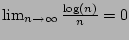, which we could prove using L'Hopital's rule.

Theorem 6.4.10 (Root Test)   Consider the sum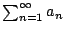.
1. If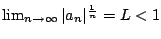, thenconvergest absolutely.
2. If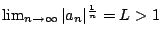, thendiverges.
3. If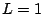, then we may conclude nothing from this!

Proof. We apply the comparison test (Theorem 6.4.1). First suppose. Then there is a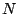such that for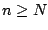we have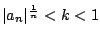. Thus for such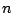we have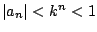. The geometric series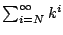converges, so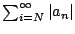also does, by Theorem 6.4.1. If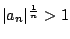for, then we see thatdiverges by comparing with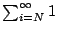.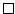Example 6.4.11   Let's apply the root test to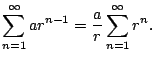We have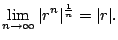Thus the root test tells us exactly what we already know about convergence of the geometry series (except when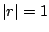).

Example 6.4.12   The sum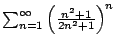is a candidate for the root test. We have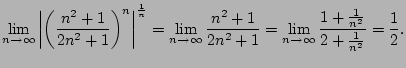Thus the series converges.

Example 6.4.13   The sum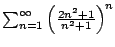is a candidate for the root test. We have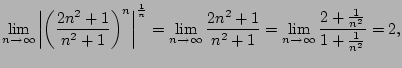hence the series diverges!

Example 6.4.14   Consider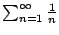. We have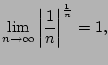so we conclude nothing!

Example 6.4.15   Consider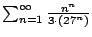. To apply the root test, we compute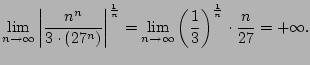Again, the limit diverges, as in Example 6.4.8.

William Stein 2006-03-15